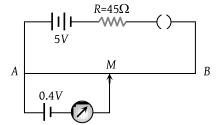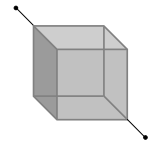The current flowing in a coil of resistance 90 Ω is to be reduced by 90%. What value of resistance should be connected in parallel with it

(1) 9 Ω

(2) 90 Ω

(3) 1000 Ω

(4) 10 Ω

High Yielding Test Series + Question Bank - NEET 2020

Difficulty Level:

A galvanometer of 50 ohm resistance has 25 divisions. A current of 4 × 10–4 ampere gives a deflection of one division. To convert this galvanometer into a voltmeter having a range of 25 volts, it should be connected with a resistance of :

(1) 2500 Ω as a shunt

(2) 2450 Ω as a shunt

(3) 2550 Ω in series

(4) 2450 Ω in series

Concept Questions :-

Meter bridge and potentiometer
High Yielding Test Series + Question Bank - NEET 2020

Difficulty Level:

In a metre bridge experiment, the null point is obtained at 20 cm from one end of the wire when resistance X is balanced against another resistance Y. If X < Y, then where will be the new position of the null point from the same end, if one decides to balance a resistance of 4X against Y

(1) 50 cm

(2) 80 cm

(3) 40 cm

(4) 70 cm

Concept Questions :-

Meter bridge and potentiometer
High Yielding Test Series + Question Bank - NEET 2020

Difficulty Level:

In given figure, the potentiometer wire AB has a resistance of 5 Ω and length 10 m. The balancing length AM for the emf of 0.4 V is :(1) 0.4 m

(2) 4 m

(3) 0.8 m

(4) 8 m

Concept Questions :-

Meter bridge and potentiometer
High Yielding Test Series + Question Bank - NEET 2020

Difficulty Level:

In a potentiometer experiment, the balancing with a cell is at length 240 cm. On shunting the cell with a resistance of 2 Ω, the balancing length becomes 120 cm. The internal resistance of the cell is :

1. 4 Ω

2. 2 Ω

3. 1 Ω

4. 0.5 Ω

Concept Questions :-

Meter bridge and potentiometer
High Yielding Test Series + Question Bank - NEET 2020

Difficulty Level:

If the resistance of voltmeter is 10000Ω and resistance of ammeter is 2Ω then find R when voltmeter reads 12V and ammeter reads 0.1 A :

(1) 118 Ω

(2) 120 Ω

(3) 124 Ω

(4) 114Ω

Concept Questions :-

Kirchoff's voltage law
High Yielding Test Series + Question Bank - NEET 2020

Difficulty Level:

Potentiometer wire of length 1 m is connected in series with 490 Ω resistance and 2V battery. If 0.2 mV/cm is the potential gradient, then the resistance of the potentiometer wire is :

(1) 4.9 Ω

(2) 7.9 Ω

(3) 5.9 Ω

(4) 6.9 Ω

Concept Questions :-

Meter bridge and potentiometer
High Yielding Test Series + Question Bank - NEET 2020

Difficulty Level:

In an electrical cable, there is a single wire of radius 9 mm of copper. Its resistance is 5 Ω. The cable is replaced by 6 different insulated copper wires, the radius of each wire is 3 mm. Now the total resistance of the cable will be :

(1) 7.5 Ω

(2) 45 Ω

(3) 90 Ω

(4) 270 Ω

Concept Questions :-

Combination of resistors
High Yielding Test Series + Question Bank - NEET 2020

Difficulty Level:

Two uniform wires A and B are of the same metal and have equal masses. The radius of wire A is twice that of wire B. The total resistance of A and B when connected in parallel is :

(1) 4 Ω when the resistance of wire A is 4.25 Ω

(2) 5 Ω when the resistance of wire A is 4.25 Ω

(3) 4 Ω when the resistance of wire B is 4.25 Ω

(4) 4 Ω when the resistance of wire B is 4.25 Ω

Concept Questions :-

Derivation of Ohm's law
High Yielding Test Series + Question Bank - NEET 2020

Difficulty Level:

Twelve wires of equal length and same cross-section are connected in the form of a cube. If the resistance of each of the wires is R, then the effective resistance between the two diagonal ends would be(1) 2R

(2) 12R

(3) $\frac{5}{6}R$

(4) 8R

Concept Questions :-

Combination of resistors